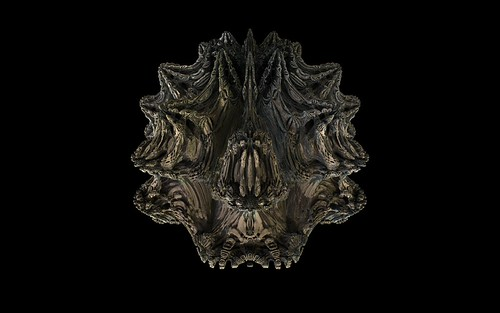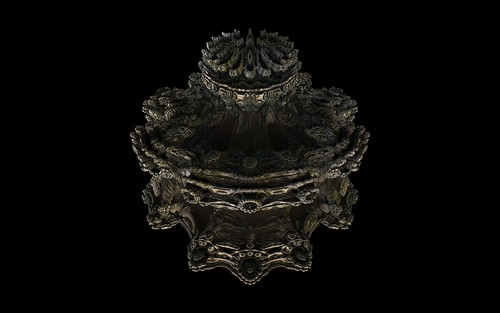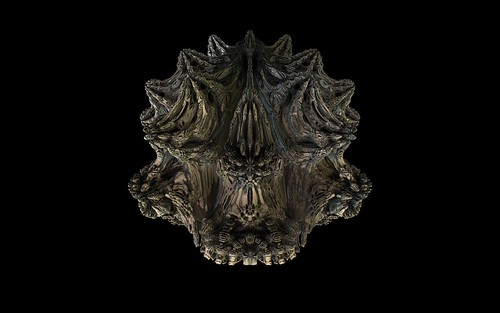# New Mandelbulb Variations

Rule 52 – Power 8 MandelbulbRule 330 – Power 8 MandelbulbRule 330 – Power 8 MandelbulbTad Boniecki emailed me with some new Mandelbulb trig variations. The only changes in these from the usual method of rendering Mandelbulbs is the formula for Triplex Power. For the original Positive SIN Mandelbulb the routine looks like;

function SINTriplexPower(const z:triplex;p:double):triplex;
var rcosphi:double;
begin
theta:=theta*p;
phi:=phi*p;
end;

The new methods changed the trig values for X, Y and Z. The main change here is there is only a single trig call for each XYZ component.

function Trig1TriplexPower(const z:triplex;p:double):triplex;
begin
theta:=theta*p;
phi:=phi*p;
end;

Theta and Phi are initialised by using

theta:=arctan2(z.y,z.x);

After randomly trying different combinations for a while, I wrote a simple loop to go through all possible combinations of these type of Mandelbulb.

For each of the XYZ components they can be in the form +/- sin/cos(phi/theta). eg -cos(phi) or sin(theta) etc. This gives a total of 512 possible combinations.

Using a Wolfram like rule numbering system, convert a nine digit binary number into decimal. The digits of the binary number correspond to;
1. 0 for X COS, 1 for X SIN
2. 0 for X Phi, 1 for X Theta
3. 0 for +, 1 for –
4. 0 for Y COS, 1 for Y SIN
5. 0 for Y Phi, 1 for Y Theta
6. 0 for +, 1 for –
7. 0 for Z COS, 1 for Z SIN
8. 0 for Z Phi, 1 for Z Theta
9. 0 for +, 1 for –
So rule number 20 is binary 000010100 and converts into the formulas

You can see all 512 variations (all power 8 Mandelbulbs) here. Each of the image names show the rule number and corresponding trig calls.

From the 512 possible results, I ignored most of them (too spikey, too similar to others, too lathed, or assymetrical) and picked the 20 most interesting results.

Rule 50Rule 52 (This is also the rule for the image at the top of this post)Rule 53Rule 59Rule 61Rule 114Rule 116Rule 117Rule 124Rule 176Rule 177Rule 188Rule 280Rule 330Rule 369Rule 370Rule 377Rule 412Rule 413Rule 465All of these new variations are now avilable in Visions Of Chaos.

Tad also had a few other new variations with more trig calls I will experiment with and explain in a future post.

Jason.